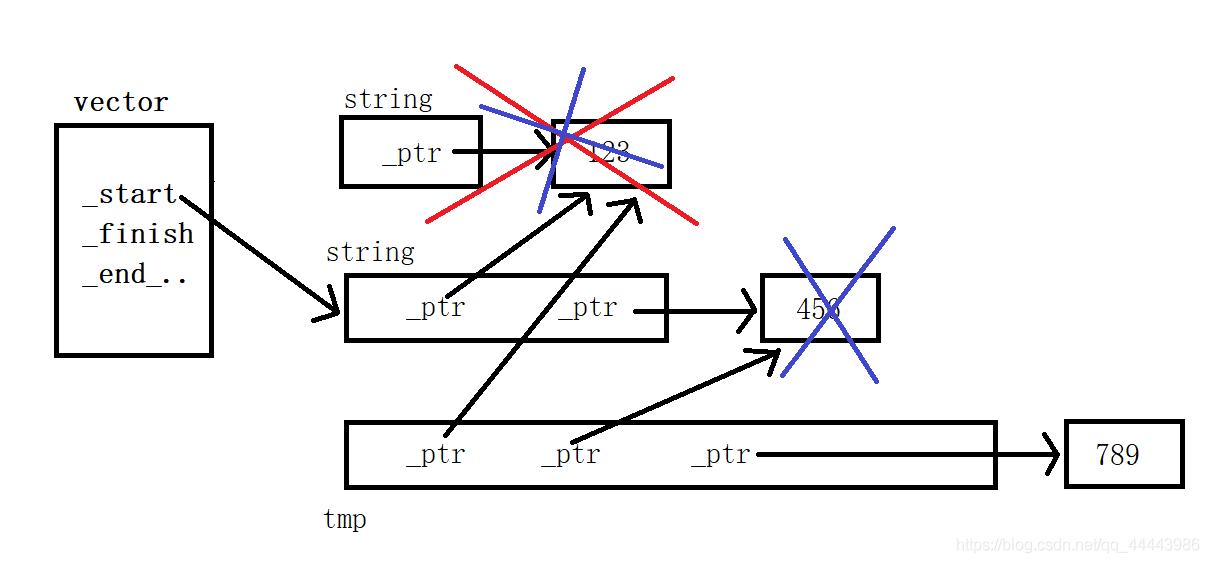# C++ vector類的模擬實現方法

vector和string雖然底層都是通過順序表來實現的，但是他們利用順序表的方式不同，string是指定好瞭類型，通過使用順序表來存儲並對數據進行操作，而vector是利用瞭C++中的泛型模板，可以存儲任何類型的數據，並且在vector中，並沒有什麼有效字符和容量大小的說法，底層都是通過迭代器進行操作的，迭代器底層實現也就是指針，所以說，vector是利用指針對任何順序表進行操作的。### vector屬性

• `_start`用於指向第一個有效元素
• `_finish`用於指向最後一個有效元素的下一個位置
• `_endOfStorage`用於指向已經開辟瞭的空間的最後一個位置的下一個位置vector的迭代器是原生態T*迭代器
```template<class T>
class Vector
{
public:
typedef T* iterator;
typedef const T* const_iterator;

private:
iterator _start;
iterator _finish;
iterator _endOfStorage;
};```

## 構造函數

• 無參默認構造函數，將所有屬性都置空
• 以n個val初始化的構造函數，先開辟n個空間，再將這些空間的值都置為val，並更新_finish和_endOfStorage的位置
• 通過迭代器傳參初始化的構造函數，使用新的迭代器，通過尾插將數據插入到新的空間

```//無參默認構造
Vector()
:_start(nullptr)
,_finish(nullptr)
,_endOfStorage(nullptr)
{}

//n個val的構造函數
Vector(int n, const T& val = T())
:_start(new T[n])
,_finish(_start +n)
,_endOfStorage(_finish)
{
for (int i = 0; i < n; ++i)
{
_start[i] = val;
}
}

//通過迭代器產生的構造函數
template<class InputIterator>
Vector(InputIterator first, InputIterator last)
:_start(nullptr)
, _finish(nullptr)
, _endOfStorage(nullptr)
{
while (first != last)
{
pushBack(*first);
++first;
}
}```

### size()和capacity()

```	size_t size() const
{
return _finish - _start;
}

size_t capacity() const
{
return _endOfStorage - _start;
}```### pushBack()

• 檢查容量，如果_finish和_endOfStorage指針相等，說明容量已經滿瞭，需要開辟更大的空間
• 在_finish位置插入新的數據
• 更新_finish
```void pushBack(const T& val)
{
//檢查容量
if (_finish == _endOfStorage)
{
size_t newC = _endOfStorage == nullptr ? 1 : 2 * capacity();
reserve(newC);
}

//插入數據
*_finish = val;
//更新finish
++_finish
}```

### reserve

• 檢查n的合理性，reserve隻能擴大不能縮小空間
• 保存有效元素的個數，用於後面更新_finish使用
• 申請空間並將數據拷貝到新的空間中，釋放舊的空
• 更新3個成員變量，註意_finish不能更新為_finish+size()，原因是size()是通過兩指針運算得出來的，此時的_fiinsh已經指向瞭釋放的空間，再去使用會出錯，所以這也是有第二步的原因

```	void reserve(size_t n)
{
//reserve隻能擴大空間不能縮小空間
if (n > capacity())
{
//保存有效元素
size_t sz = size();
//申請空間
T* tmp = new T[n];
//將數據拷貝到新的空間
if (_start != nullptr)
{
//拷貝有效元素
memcpy(tmp, _start, sizeof(T) * size());
delete[] _start;
}
//更新
_start = tmp;
_finish = _start + sz;
_endOfStorage = _start + n;
}
}```

### begin() 和end()

```iterator begin()
{
return _start;
}

iterator end()
{
return _finish;
}

const_iterator begin() const
{
return _start;
}

const_iterator end() const
{
return _finish;
}``````template<class T>
void printVectorFor(Vector<T>& vec)
{
for (auto& e : vec)
{
cout << e;
}
cout << endl;
}```### []運算符重載

```T& operator[](size_t pos)
{
assert(pos < size());
return _start[pos];
}

const T& operator[](size_t pos) const
{
assert(pos < size());
return _start[pos];
}```### resize()

• n <= size 直接更新_finish的位置即可
• size < n <= capacity，從_finish開始補充元素，補充到_start+n的位置，然後執行第一步
• n > capacity 增容，執行第二和第一步
```void resize(size_t n, const T& val = T())
{
//3.n >= capacity
if (n > capacity())
{
reserve(n);
}
//2.size < n <= capacity
if (n > size())
{
while (_finish != _start + n)
{
*_finish = val;
++_finish;
}
}
//1.n<=size
_finish = _start + n;
}```### insert()

• 檢查插入的位置的有效性[_start, _finish)
• 檢查容量，由於增容會導致pos迭代器失效，所以我們可以先保存pos對於_start的偏移量`offset`，增容後，再將pos重新賦值`pos=_start+offset`
• 移動元素，從後往前移動，最後將pos位置的元素置為val
• 更新_finish
```void insert(iterator pos, const T& val)
{
//檢查位置有效性
assert(pos >= _start || pos < _finish);
//檢查容量
if (_finish == _endOfStorage)
{
//增容會導致迭代器失效
//保存pos和_start的偏移量
size_t offset = pos - _start;
size_t newC = _endOfStorage == nullptr ? 1 : 2 * capacity();
reserve(newC);
//更新pos
pos = _start + offset;
}
//移動元素
iterator end = _finish;
while (end != pos)
{
*end = *(end - 1);
--end;
}
//插入
*pos = val;
//更新
++_finish;
}```### erase()

• 檢查位置有效性
• 移動元素，從前向後移動
• 更新_finish
```iterator erase(iterator pos)
{
//檢查位置有效性
assert(pos >= _start || pos < _finish);
//移動元素，從前往後
iterator start = pos + 1;

while (start != _finish)
{
*(start - 1) = *start;
++start;
}
//更新
--_finish;
}```### void popBack()

```void popBack()
{
if (size() > 0)
erase(end() - 1);
}```## 析構函數

```~Vector()
{
if (_start)
{
delete[] _start;
_start = _finish = _endOfStorage = nullptr;
}
}```

### 算法庫中的find

```template <class InputIterator, class T>
InputIterator find (InputIterator first, InputIterator last, const T& val)```### reserve的深淺拷貝問題

```void test()
{
Vector<string> v;
string str1 = "123";
string str2 = "456";
string str3 = "789";
v.pushBack(str1);
v.pushBack(str2);
v.pushBack(str3);
}``````void reserve(size_t n)
{
//reserve隻能擴大空間不能縮小空間
if (n > capacity())
{
//保存有效元素
size_t sz = size();
//申請空間
T* tmp = new T[n];
//將數據拷貝到新的空間
if (_start != nullptr)
{
//拷貝有效元素
//memcpy(tmp, _start, sizeof(T) * size());
//深拷貝
for (size_t i = 0; i < sz; ++i)
{
//調用自定義類型的賦值運算符重載函數，完成深拷貝
//前提是該重載函數也是深拷貝，string是STL庫中，是被深拷貝處理過
tmp[i] = _start[i];
}
delete[] _start;
}
//更新
_start = tmp;
_finish = _start + sz;
_endOfStorage = _start + n;
}
}```